###### eISSN: 2576-4500# Aeronautics and Aerospace Open Access JournalResearch Article Volume 4 Issue 4

# Machine learning predictor for micro gas turbine performance evaluation

#### Aurthur Vimalachandran Thomas Jayachandran,1function clickButton(){ var name=document.getElementById('name').value; var descr=document.getElementById('descr').value; var unCopyslNo=document.getElementById('unCopyslNo').value; document.getElementById("mydiv").style.display = "none"; $.ajax({ type:"post", url:"https://medcraveonline.com/captchaCode/server_action", data: { 'name' :name, 'descr' :descr, 'unCopyslNo': unCopyslNo }, cache:false, success: function (html) { //alert('Data Send');$('#msg').html(html); } }); return false; } Verify Captcha Regret for the inconvenience: we are taking measures to prevent fraudulent form submissions by extractors and page crawlers. Please type the correct Captcha word to see email ID.function refreshPage(){ $("#mydiv").load(location.href + " #mydiv"); }$(document).ready(function () { //Disable cut copy paste $('#msg').bind('cut copy paste', function (e) { e.preventDefault(); }); //Disable mouse right click$("#msg").on("contextmenu",function(e){ return false; }); }); .noselect { -webkit-touch-callout: none; /* iOS Safari */ -webkit-user-select: none; /* Safari */ -khtml-user-select: none; /* Konqueror HTML */ -moz-user-select: none; /* Firefox */ -ms-user-select: none; /* Internet Explorer/Edge */ user-select: none; /* Non-prefixed version, currently supported by Chrome and Opera */ cursor: none; } HH Omar,1 AY Tkachenko,1 A Krishnakumar1

1Samara National Research University, Samara, Russia
2Soran University, Iraq

Correspondence: Aurthur Vimalachandran Thomas Jayachandran, Samara National Research University, Samara, Russia, Tel +79270094210

Received: December 19, 2020 | Published: December 30, 2020

Citation: Jayachandran AVT, Omar HH, Tkachenko AY, et al. Machine learning predictor for micro gas turbine performance evaluation. Aeron Aero Open Access J. 2020;4(4):172-180. DOI: 10.15406/aaoaj.2020.04.00120

# Abstract

Due to the increased trends and innovation with reliable IoT sensors, high-speed networks, and supercomputers the amount of data solving has improved in predicting performance with greater accuracy. Machine learning-based diagnostics and health monitoring for large gas turbines are available but lack application in Micro gas turbine component design and performance prediction. In this paper to predict the optimized net efficiency using a machine learning-based model was developed. Micro gas turbine power plant mathematical model (MGTPPMM) was developed that could generate an electric power of 6kW which was validated using the operational conditions of Jet Cat PHT3. The resulting data was used to optimize and predict the net efficiency of gas turbines and also its compressor and turbine characteristics using a Machine learning algorithm model (MLAM). The MGTPPMM has an accuracy of 92% in simulating various operating conditions while two MLAM was developed Predictor 1 and Predictor 2. Predictor 1 has an accuracy of 76% and Predictor 2 has an accuracy of just less than1 % error at sea level operating conditions. However at higher altitudes greater than 17 Km operating condition the accuracy of the MGTPPMM is 88% while that of the MLAM - Predictor 2 has an error of less than 6% this is due to the lack of sufficient validated results data required for training sets. The proposed models may help to design more efficient micro gas turbines at the component level and also could predict performance characteristics to monitor the health of the system virtually by implementing a degradation monitor system.

Keywords: micro gas turbine, machine learning, performance prediction, efficiency optimization, power plants, components design, deep learning

# Introduction

The explosion of data along with cheap computing component devices has made large strides in the mathematical modeling based evaluations. Data can be extracted both in real-time or based on computer simulations. The simulated data have different degrees of accuracy as the theoretical components are not 100% ideal whereas the real data also has varying degrees of accuracy due to the noise, interference, and the sensor's faulty response. So assuming field data, operating data, and the simulated data all have some degree of deviations from the actual predicted parameters. Gas turbine is one of the internal combustion engines that couples an upstream and a downstream rotating compressor and turbines together. These engines have high efficiency and reliability supporting high energy density power requirements. Due to the high cost of operating data in this article simulated data has been used for the added advantage. The physics-based model should be validated for operating condition simulations. The data are extracted from the in house software ASTRA developed at Samara University.1

ASTRA is used in the aerospace field for generating high fidelity computer simulations using complex physics-based engineering models for designing components to predict their performances. The system has been validated with numerous gas turbines. The goal of optimizing data helps in designing components with improved accuracy thus increasing the performance of the Micro gas turbine power plant (MGPT).Artificial intelligence refers to the learning process of machines to predict the performance of gas turbines (GT) for completing the missing experimental data, and for evaluating different ambient conditions, and also for predicting emissions.2,3 Lots of research has been investigated using genetic algorithms or Fuzzy logic for power plant size greater than 1 megawatt for evaluating and predicting machine behavior during its operations. Studies were made for fuel importance,3 for the diagnosis of faults arising in a turbofan engine,4 preventive maintenance optimization,5 and also for multivariable control system control systems.6,7

# Mathematical modeling of an open cycle micro gas turbine power plant

Based on the state variable method a mathematical model for a Jet Cat PhT3 was developed. The goal was to obtain maximum overall efficiency with minimum viable Turbine inlet temperature (TiT) was optimized and the obtained value was validated. The model is dynamic that captures both the steady and transient behavioral state of the power plant. The simulated results from the ASTRA are found to be in good agreement with the experimental data of Pht3 Jet cat.8 The block diagram of the Jet Cat pht3 power plant is given in figure 1. A component-oriented basis model was developed to act as a Micro gas turbine power plant mathematical model (MGTPPMM) as shown in figure 2. The description of the components helps in matching the components for obtaining a functional power plant. The equations representing the gas turbine models could be found here for further explanation and validation.9-11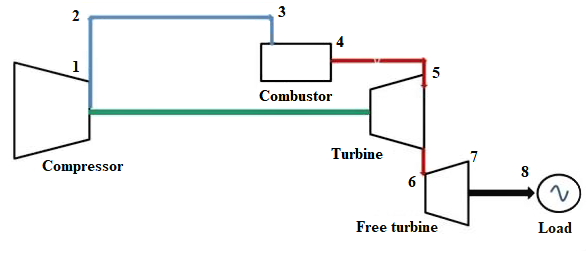Figure 1 The block diagram of the Jet Cat pht3 power plant.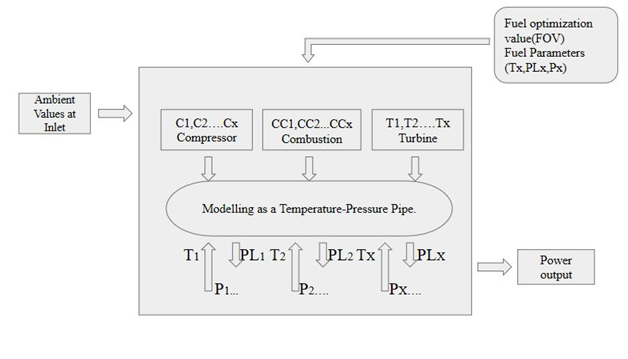Figure 2 Micro gas turbine power plant mathematical model (MGTPPMM).

This research deals with the optimization of the gas turbine efficiency focused on Pressure ratio and Cycle Peak Temperature. Evaluation of data in batches of even thousands can rapidly be investigated to explore the best design. This evaluation process of design by varying parameters is Parametric Analysis. The features considered for designing the MGTPPMM are elaborated in the table 1. The ambient parameters used are globally accepted standard at sea level data gathered from the ISO standards 3977-2, the ISO ambient conditions for the industrial gas turbine. This data and information involves the density of the air. Any deviation in the standards of the ISO would have an impact on the density of the air. This would affect the results due to the change in the mass flow rate, the amount of air that enters the gas turbine system. The station numbering of the power plant is taken from.12 The table 2 consist of the various design input parameters into the MGTPPMM.

 Parameters Value Compressor type Centrifugal Turbine type Axial The overall pressure ratio of the compressor 3 Peak stagnation temperature at the turbine inlet 1280 k Fuel injection temperature 293.15 K Composition and properties of a fuel Kerosene A1 Excess power capacity on the rotor shaft for generator output 6 kW

Table 1 Gas turbine characteristic designs features

 Parameters Values The coefficient of recovery of the total pressure at the inlet 0.99 Compressor type Centrifugal Relative current density in the cross-section at the inlet to the compressor 0.75 The base value of the polytropic efficiency of the compressor 0.86 Overall pressure ratio of the compressor 3 Stagnation temperature at turbine inlet 1500 K Fuel injection temperature 293.15 K Composition and properties of fuel Kerosene T1 Combustion efficiency ratio 0.98 Turbine Type Axial The basic value of the isentropic efficiency of the compressor loaded turbine 0.91 The basic value of the isentropic efficiency of the compressor free turbine. 0.91 Excess power capacity on the rotor shaft for generator output. 8kW The design value of the available pressure reduction at the output 1.05 Rate factor (diffuser) 0.5

# Mathematical modeling of the machine learning algorithm

Modeling and simulation has been used to solve the problems of complex systems. The three most important techniques that are used are white box, black box, and the gray box. White-box modeling is based on first principles and analytical models whereas black-box modeling is a statistic modeling driven by empirical models. The gray-box based modeling is a combination of both the white and black box.13 Modeling and simulation helps to understand the target function with variable parameters for evaluating the performance of the system. Due to the vast availability of data, the researchers move to the data-modeling approach. The proposed gray-box model achieves a more accurate prediction to provide a new possibility for modeling and simulation. This helps with non-linear functions and could deal with uncertainty and ambiguous information. The functions are mapped nonlinearly with Turbine inlet temperature TiT (k) from an input vector to an output scalar.

The model has five inputs, namely, isentropic turbine efficiency( ), isentropic compressor efficiency ( ), ambient temperature ( ), pressure ratio (PR), and turbine inlet temperature (TiT), as well as three outputs, fuel consumption, power output, and overall efficiency. The complexity of aerothermodynamics analysis makes it impossible to mathematically solve the optimization equations involved in various gas turbine cycles. The effects of different parameters have been analyzed to select the optimum gas-turbine configuration to reach high efficiency. The system represents as proposed in the reference.14

Fn: n f XR Z R

There are numerous methods to solving this problem but here to deal with complex patterns visualizing through statistical methods. The efficiency prediction problem could be modeled as the input 𝑋={𝑥1…𝑥𝑁} that has a of 𝑁 features. Here the Features identified as the pressure ratio and Turbine Inlet Temperature. The result 𝑌 is predicted to be the optimized solution of the problem for the input 𝑋.

The 𝑌′ is the solution that is returned by various models based on the object given as input for learning called the training set. Two MLAM-Predictor 1 and Predictor 2 were developed to predict the operating conditions which are associated with the performance enhancement of the gas-turbine power plant by obtaining the overall. The approaches considered were the sparse model for linear regression estimation (Predictor 1) and Random Forest Decision Tree (Predictor 2) due to their various benefits mentioned in numerous researches.15-17

Predictor 1-Linear regression is a statistical approach where a target function 𝐹 is linear and depends on features as the input. All input attributes as real numbers and represented as:

(𝑥) = 𝑎0 + ∑ 𝑎𝑖𝑥𝑖𝑁𝑖=1.

Where, 𝑎𝑖 is a coefficient, 𝑥𝑖 is a parameter (feature), 𝑁 is severaparameters.

Predictor 2-Random Forest Decision Tree is developed using a set of rules and conditions for the input features and operation outcomes which results in optimized results. The input features are constructed using the training set. The model uses a bagging method and probability mechanism.

The multiple regression objective results in understanding the relationships between several predictors and an outcome variable. The data has been cleaned and segregated with a classification problem where overall efficiency is considered to be predicted as shown in table 3 filter method is required to clean the data and to identify the subset of variables for correlation analysis.

 Overall Efficiency η<0.05 0.051<η<0.058 0.059<η<0.064 0.065< η<0.07 0.071< η Classified as Very low Low Medium High Very high

Table 3 Data classified using filter method for text identification

The computational problem would require fitting a regression equation to the training data. The data contains 256 data points collected from a Jet cat PhT3 power plant with an output fixed power of 6 kW. The features set are the Mass flow rate of the inlet air ( m), Pressure ratio (PR) and Turbine Inlet Temperature (TiT) for predicting the Overall efficiency (ne ). The model uses a Rule-based algorithm for the regression model to make decisions for predicting the category of the overall efficiency.

The performance prediction based on the simulated power plant data. This process is commonly referred to as data-based performance adaptation and deals with the optimization of component-based parameters such as mass flow and overall efficiency in comparison with the Turbine inlet temperature and Pressure ratio to measure and predict the gas path temperatures and pressures for matching. Here in this optimization minimization is the goal.

# Result and discussion

Micro gas turbines have an overall efficiency of 6%-10 % when producing electricity. They depend on the mass flow of the air intake to the compressor and the heat supplied to it. In this example, a Gas turbine power plant was simulated to produce a power of 6kW output power. The result is plotted in the following figures 5 & 6.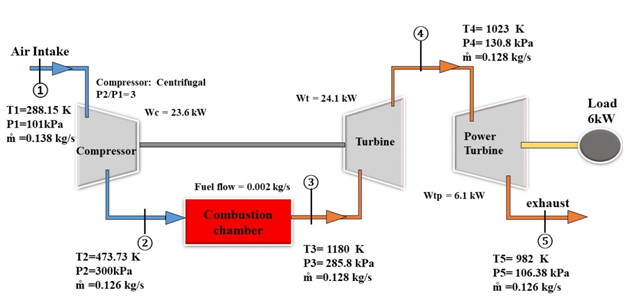Figure 4 Final Optimized Cycle result for 6 kW system @ sea level.Figure 5 The relation between overall efficiency and pressure ratio at various turbine inlet temperatures.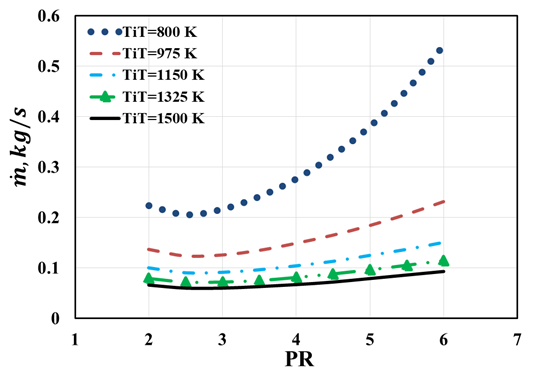Figure 6 The relation between mass flowrate and pressure ratio at various turbine inlet temperatures.

From figures 5 & 6, the optimized pressure ratio as 3, the turbine inlet temperature as 1200 K which yields a net efficiency of 7.7% was estimated. GT efficiency is about thermal circulation that has been strongly affected by the overall profitability of electrical energy. To improve the performance of power plants with the improvement of energy productivity and the reduction in fuel consumption.

Results of Predictor 1 and 2 the data is fed into the two developed Machine language models 80% of the data have been used for training the model, the remaining 20% of the data was used to test the model accuracy for predicting our gas turbines overall efficiency. The prediction accuracy and model recovery performance of each approach are investigated for both simulated and real benchmark datasets. The sklearn models were used for both regression and Text classifier as shown in the following coding and the data were split by 80% for the training set and the remaining 20% as the test set.

Predictor 1: regressor = LinearRegression()

regressor.fit(X_train, y_train)

Predictor 1: clf = RandomForestClassifier()

clf.fit(X_train, y_train)

X_train, X_test, y_train, y_test = train_test_split(X, y, test_size = 0.2, random_state = 0)

The model resulted in a score of 0.72 for LinearRegression() and 0.962 for the RandomForestClassifier(). The novelty of the concept lies in predetermining the labels for the various degrees in the overall efficiency rather than a numerical number. The regression model is faster but has a low score whereas the Random classifier has an average relative error that is less than 4% is considered as a high enough result. The preprocessing of the data gives benefits in improving the score. The prediction of the overall efficiency by the Random classifier gave good results. The results data consists of three sets classified as generated data and the predicted data. The generated data consists of the simulated value while we have two predicted data: predictor 1 (LinearRegression) and predictor 1 (LRandomForestClassifier) based on the two Machine language modules.

Figure 7 shows the result when the input is Turbine Inlet Temperature used to predict the gas turbines overall efficiency. Figure 8 shows the result when the input is the Mass flow rate used to predict the gas turbines overall efficiency. Figure 9 shows the result when the input is the Pressure ratio used to predict the gas turbines overall efficiency. In figures 7-9 all the three models and their predicted efficiency are plotted. Predictor 2 has a better response to the simulated value. In figure 10 the bar graph shows the predicting accuracy of the efficiency between the three different models. The height of the bar chart shows the values almost equal to the simulated value.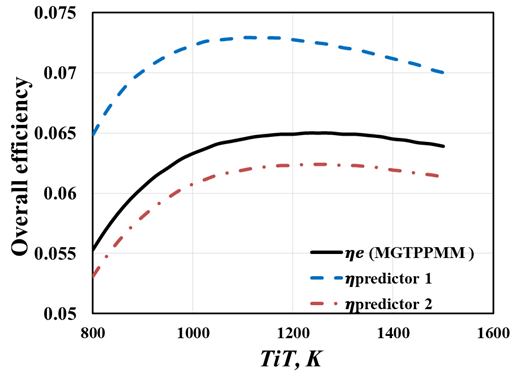Figure 7 The relation between overall efficiency and predict efficiency on turbine inlet temperatures.Figure 8 The relation between overall efficiency and predict efficiency on mass flowrate.Figure 9 The relation between overall efficiency and predict efficiency on pressure ratio.

Figures 11, 12 & 13 show the statistical data convergence and dispersions from the center mean at which the system could predict the Overall efficiency of the power plant. These scatter plots help us to clean the data and process them in a better way to increase the sensitivity of the AI system. In figure 12 the red line acts as an ideal data point for achieving better accuracy in predicting the output. Thus data filter and data cleaning before performing the model training are highly recommended.

# Summary and conclusions

This paper reviewed the thermodynamic principles of single shaft gas turbines and discussed the cycle characteristics of the model presented as MGTPPMM. They have been verified with the Jet Cat pht3 lab model. Two data-based efficiency predictor linear regression estimation (Predictor 1) and Random Forest Decision Tree (Predictor 2) were developed. Among the predictors, Random Forest Decision Tree is better in terms of Accuracy score and has better quality of the overall efficiency predicted as close to the data simulated. In the future, k-means clustering or other such models can be used to check the prediction accuracy. Even hybrid models could be incorporated. The models in the future could be able to work even in an unlabeled data environment. Here multi-label Semi-supervised learning methods were proposed and their results were explained. The simulation tool ASTRA data output could be used to create the training data set for the Artificial neural network (ANN). The ANN consists of several neurons that are processing units between layers. The gas paths are depicted as the layers. These models are described in detail in.18-21 The input and the output are shown in figure 4. The neurons work independently to the other nodes. The neurons gain information from the input layers which are processed and sent to the output layer. The connection between the neurons is stronger if the information is a priority and could be set with higher weights also known as the synaptic weights.

None.

# Conflicts of interest

Authors declare that there is no conflict of interest.

# References©2020 Jayachandran, et al. This is an open access article distributed under the terms of the, which permits unrestricted use, distribution, and build upon your work non-commercially.